```
Stewart Dickson
110 N. Whipple St.
Fort Bragg, CA  95437
(707)813-0385
MathArtSPD@GMail.com
```
Contact: The Williams Gallery.

# Portfolio of Fermat's Last Theorem

(updated 28 June, 1999)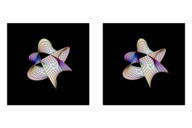Complex Projective Varieties Determined by x^3 + y^3 = z^3 (Fermat's Last Theorem), n = 3, Stereoscopic Mathematica computer rendering, Harvest Fine Art Print on archival paper, (c) 1990 Andrew Hanson, Stewart Dickson.Complex Projective Varieties Determined by x^3 + y^3 = z^3 (Fermat's Last Theorem), n = 3. 8.5" X 6.0" X 6.0", Stereolithograph, (c) 1991 Andrew Hanson, Stewart Dickson.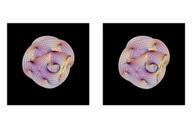Complex Projective Varieties Determined by x^5 + y^5 = Z^5 (Fermat's Last Theorem), n = 5, Stereoscopic Mathematica computer rendering, Harvest Fine Art Print on archival paper, (c) 1990 Andrew Hanson, Stewart Dickson.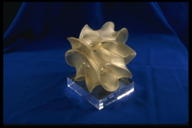Complex Projective Varieties Determined by x^5 + y^5 = Z^5 (Fermat's Last Theorem), n = 5. 9.0" X 6.8" X 6.8", Stereolithograph, (c) 1991 Andrew Hanson, Stewart Dickson.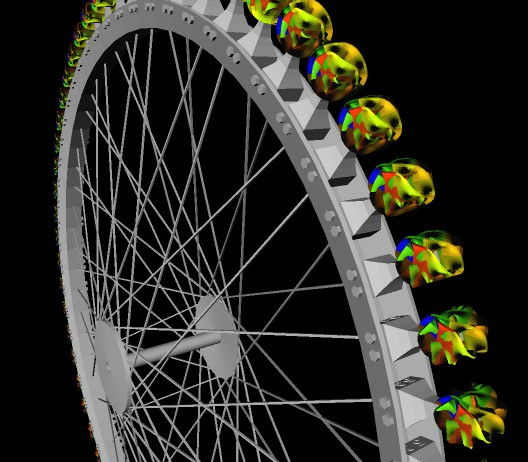A 3-D Zoetrope, Computer-Rendered Proposal.Complex Projective Varieties Determined by x^7 + y^7 = Z^7 (Fermat's Last Theorem), n = 7, Mathematica computer rendering, (c) 1990 Andrew Hanson, Stewart Dickson.Complex Projective Varieties Determined by x^9 + y^9 = Z^9 (Fermat's Last Theorem), n = 9, Mathematica computer rendering, (c) 1990 Andrew Hanson, Stewart Dickson.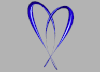Press Here to go to Mathart.com home page.Press Here to go to Stewart Dickson's General Portfolio